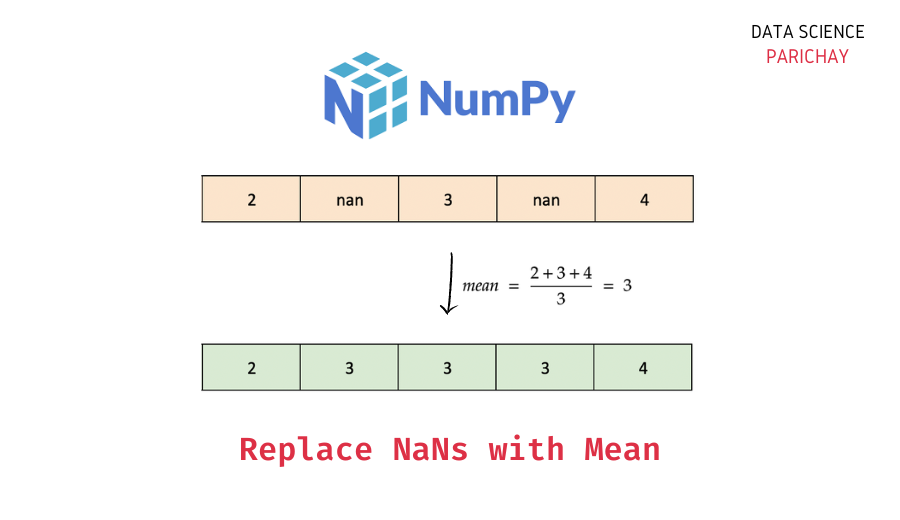# Numpy – Replace NaN Values with Mean

In this tutorial, we will look at how to replace all occurrences of NaN values in a Numpy array with the mean value in the array with the help of some examples.

## How do I replace all NaN values with the mean in Numpy?

Use boolean indexing to replace all instances of NaN in a Numpy array with the mean. Here, we use the `numpy.isnan()` function to check whether a value inside the array is NaN or not, and if it is, we set it to the mean value.

The following is the syntax –

```import numpy as np
ar[np.isnan(ar)] = np.nanmean(ar)```

Use the `numpy.nanmean()` function to compute the mean of a Numpy array containing NaN values. It calculates the mean excluding the NaN values in the array.

Let’s now look at a step-by-step example of using the above syntax on a Numpy array.

### Step 1 – Create a Numpy array

First, we will create a one-dimensional array that we will be using throughout this tutorial.

```import numpy as np

# create numpy array
ar = np.array([1, 2, np.nan, 3, 4, np.nan, np.nan, 5])
# display the array
ar```

Output:

`array([ 1.,  2., nan,  3.,  4., nan, nan,  5.])`

Here, we used the `np.array()` function to create a Numpy array with some numbers and some NaN values.

📚 Data Science Programs By Skill Level

Introductory

Intermediate ⭐⭐⭐

🔎 Find Data Science Programs 👨‍💻 111,889 already enrolled

Disclaimer: Data Science Parichay is reader supported. When you purchase a course through a link on this site, we may earn a small commission at no additional cost to you. Earned commissions help support this website and its team of writers.

### Step 2 – Set NaN values in the array to the mean using boolean indexing

Use the `numpy.isnan()` function to check whether a value in the array is NaN or not. If it is, set it to the mean value (use the `numpy.nanmean()` function to get the mean of a Numpy array with NaN values).

Let’s replace all occurrences of NaN in the above array with the mean value of the array.

```# replace nan with the mean
ar[np.isnan(ar)] = np.nanmean(ar)
# display the array
ar```

Output:

`array([1., 2., 3., 3., 4., 3., 3., 5.])`

You can see that each instance of NaN has been replaced by 3. (which is the mean value in the array). Note that here we are modifying the original array.

You can also use this method to replace NaN values with the mean in higher-dimensional arrays. For example, let’s apply this method to a two-dimensional array containing some NaN values.

```# create a 2D numpy array
ar = np.array([
[1, np.nan, 2],
[np.nan, 3, 4],
[5, np.nan, np.nan]
])
# display the array
ar```

Output:

```array([[ 1., nan,  2.],
[nan,  3.,  4.],
[ 5., nan, nan]])```

Here, we created a 2D Numpy array containing some NaN values.

Let’s now replace the NaN values in this 2D array with the overall mean of the values in the 2D array.

```# replace nan with the mean
ar[np.isnan(ar)] = np.nanmean(ar)
# display the array
ar```

Output:

```array([[1., 3., 2.],
[3., 3., 4.],
[5., 3., 3.]])```

The array now has the mean (3.0) in place of NaNs.

You can similarly use this method to replace NaN values in a Numpy array with any other value.

## Summary – Replace NaN values in Numpy array with the mean

In this tutorial, we looked at how to replace all NaN values in a Numpy array with the mean value. The following is a short summary of the steps mentioned in this tutorial.

1. Create a Numpy array (skip this step if you already have an array to operate on).
2. Use the `numpy.isnan()` function to check whether a value in the array is NaN or not. If it is, set it to the mean value in the array using boolean indexing `ar[np.isnan(ar)] = np.nanmean(ar)`

You might also be interested in –

•